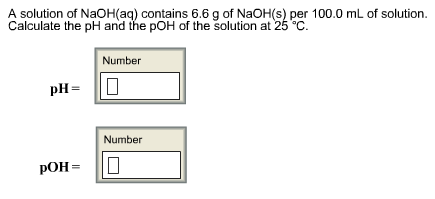# Problem: A solution of NaOH(aq) contains 6.6 g of NaOH(s) per 100.0 mL of solution. Calculate the pH and the pOH of the solution at 25°C. pH = pOH =

###### FREE Expert Solution
81% (333 ratings)###### Problem Details

A solution of NaOH(aq) contains 6.6 g of NaOH(s) per 100.0 mL of solution. Calculate the pH and the pOH of the solution at 25°C.

pH =

pOH =